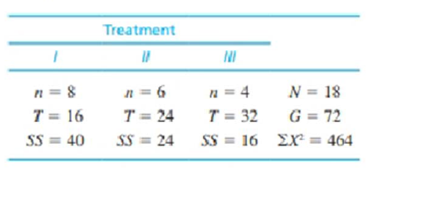Chapter 12, Problem 19PEssentials of Statistics for the B...

8th Edition
Frederick J Gravetter + 1 other
ISBN: 9781133956570

Solutions

Chapter
SectionEssentials of Statistics for the B...

8th Edition
Frederick J Gravetter + 1 other
ISBN: 9781133956570
Textbook Problem

The following data were obtained from an independent-measures research study comparing three treatment conditions. Use an ANOVA with α = .05 to determine whether there are any significant mean differences among the treatments.To determine
Whether the treatments are significant.

Explanation

Given info:

The data represents the measures of the research study.

Calculation:

Here, k represents the numbers of treatments. Then,

k=3

Degrees of Freedom:

dfbetween=k1=31=2

dfwithin=Nk=183=15

SSwithin is obtained as:

SSwithin=SS=40+24+16=80

Sum of squares between treatments is obtained by:

SSbetween=T2nG2N=(16)28

Still sussing out bartleby?

Check out a sample textbook solution.

See a sample solution

The Solution to Your Study Problems

Bartleby provides explanations to thousands of textbook problems written by our experts, many with advanced degrees!

Get Started

3. Compute .

Mathematical Applications for the Management, Life, and Social Sciences

In Exercises 1320, plot the point on a set of coordinate axes. (4.5,4.5)

Finite Mathematics for the Managerial, Life, and Social Sciences

True or False: is a convergent series.

Study Guide for Stewart's Multivariable Calculus, 8th